## Geometric Transformation Types for Control Point Registration

The `fitgeotform2d` function can infer the parameters from control point pairs for the following types of geometric transformations, listed in order of complexity.

Transformation Type

DescriptionMinimum Number of Control Point PairsExample
`"similarity"`Use this transformation when shapes in the moving image are unchanged, but the image is distorted by some combination of translation, rotation, and isotropic scaling. Straight lines remain straight, and parallel lines are still parallel.2`"reflectivesimilarity"`Same as `"similarity"` with the addition of optional reflection.3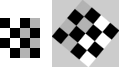`"affine"`Use this transformation when shapes in the moving image exhibit shearing. Straight lines remain straight, and parallel lines remain parallel, but rectangles become parallelograms.3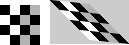`"projective"`Use this transformation when the scene appears tilted. Straight lines remain straight, but parallel lines converge toward a vanishing point.4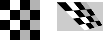`"polynomial"`Use this transformation when objects in the image are curved. The higher the order of the polynomial, the better the fit, but the result can contain more curves than the fixed image.

6 (order 2)

10 (order 3)

15 (order 4)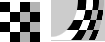`"pwl"`Use this transformation (piecewise linear) when parts of the image appear distorted differently.4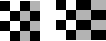`"lwm"`Use this transformation (local weighted mean), when the distortion varies locally and piecewise linear is not sufficient. 6 (12 recommended)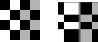The first five transformations, `"similarity"`, `"reflectivesimilarity"`, `"affine"`, `"projective"`, and `"polynomial"`, are global transformations. In these transformations, a single mathematical expression applies to an entire image. The last two transformations, `"pwl"` (piecewise linear) and `"lwm"` (local weighted mean), are local transformations. In these transformations, different mathematical expressions apply to different regions within an image. When exploring how different transformations affect the images you are working with, try the global transformations first. If these transformations are not satisfactory, try the local transformations: the piecewise linear transformation first, and then the local weighted mean transformation.

Your choice of transformation type affects the number of control point pairs you must select. For example, a similarity transformation without reflection requires at least two control point pairs. A fourth order polynomial transformation requires 15 control point pairs. For more information about these transformation types, and the special syntaxes they require, see `cpselect`.